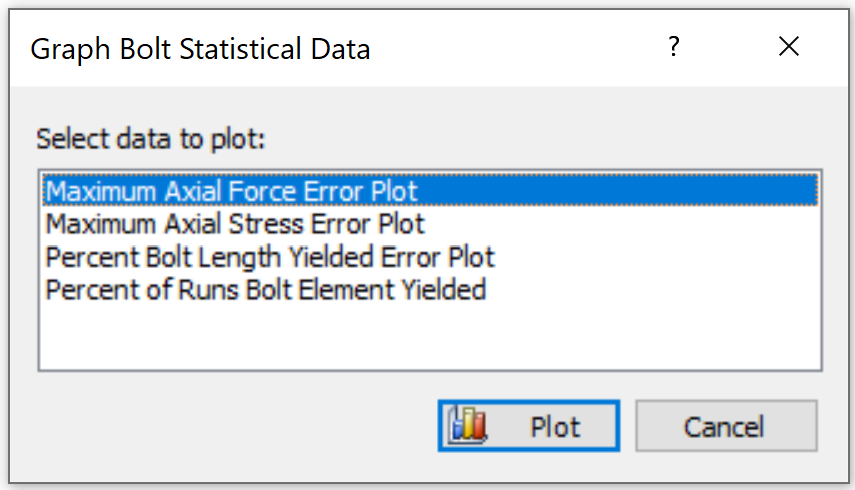# Graph Statistical Bolt Data

In RS2 Interpret, the Graph Bolt Statistical Data option allow you to plot statistical bolt data after a probabilistic analysis. This option is only available if you have computed a Probabilistic Analysis with bolts.

Four types of statistical bolt data plots are available in RS2:

1. Maximum Axial Force Error Plot – Max axial force vs. Bolt number
2. Maximum Axial Stress Error Plot – Max axial stress vs. Bolt number
3. Percent Bolt Length Yielded Error Plot – % of bolt length yielded vs. Bolt number
4. Percent of Runs Bolt Element Yielded – % of runs bolts element yielded vs. Distance

To generate a statistical bolt data plot:

1. To enable this option, make sure the files has been computed with a probabilistic analysis, and bolts are modeled.
2. Select Graph Statistical Bolt Data from the Statistics menu. A dialog will be prompted as below.
3.4. In the Graph Bolt Statistical Data dialog, select a data type to plot.
5. Select Plot when complete selection. The graph will be generated and displayed in a new window.